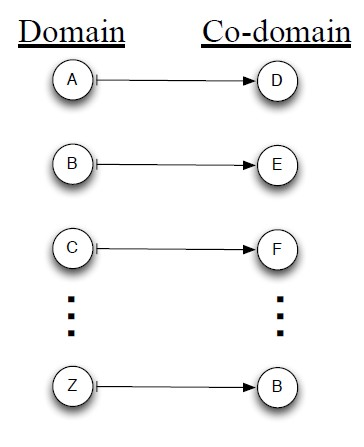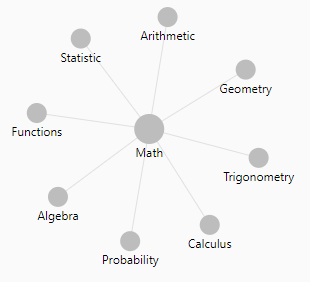# Mathematics - codomain of a function (set of output)

In mathematics, the codomain or target set of a function is the set Y into which all of the output of the function is constrained to fall.

It is the set Y in the notation f: X → Y.

X is the domain.

## Documentation / Reference

Recommended PagesLinear Algebra - Function (Set)

For each input element in a set , a function assigns a single output element from another set . is called the domain of the function is called the co-domain or in Mathese: The function...Mathematics - Domain of a function (set of input )

In mathematics, the domain of definition or simply the domain of a function is the set of “input” or argument values for which the function is defined. That is, the function provides an “output”...